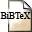# The majorization approach to multidimensional scaling for Minkowski distances

## Authors

P. Groenen, R. Mathar, W. Heiser,

## Abstract

The majorization method for multidimensional scaling with Kruskal's stress has been limited to Euclidean distances only. Here we extend the majorization algorithm to deal with Minkowski distances with 1 <= p <= 2 and suggest an algorithm that is partially based on majorization for p outside this range. We give some convergence proofs and extend the zero distance theorem of De Leeuw (1984) to Minkowski distances with p > 1.

## BibTEX Reference Entry```@article{GrMaHe95,
author = {Patrick Groenen and Rudolf Mathar and Willem Heiser},
title = "The majorization approach to {M}ultidimensional {S}caling for {M}inkowski distances",
pages = "3-19",
journal = "Journal of Classification",
volume = "12",
number = "1",
year = 1995,
hsb = RWTH-CONV-223142,
}
```Download bibtex-file